# Atmospheric correction

Aim of this tutorial:

• Transforming a Landsat ETM+ image from DN values to radiance values
• Conducting an atmospheric correction of a Landsat image using the i.atcorr module in GRASS GIS and convert radiance to reflectance values

## Introduction

Standard steps for pre-processing satellite images are:

1. Converting Digital Numbers (DN) to Top-of-Atmosphere Radiances (ToAR)
• for Landsat imagery, apply the equation below or use the i.landsat.toar module
• DNs -- which are actually the pixel values -- are the result of the quantified amount of energy observed and measured at the sensor
• the i.landsat.toar module supports all Landsat sensors icluding MSS, TM and ETM+
2. Converting ToA Radiances to Top-of-Canopy Reflectances (ToCR) → use the i.atcorr module
• the conversion to Reflectances attempts to assess for and remove atmospheric effects, a process known as atmospheric correction
• the atmospheric correction step is quite a common topic of discussion in Remote Sensing. However, depending on the quality of the acquisition(s), it can be a complex process that does not always offer a great benefit, in terms of accuracy for subsequent processing steps (e.g. image segmentation and classification).

Remember to check if water areas are represented by values >0, since reflectance is always positive. If you encounter negative reflectance values, you have a "cornichon" (pickle in English ;-0). This means that the transformation equations used, do not correspond to the image processed.

An overview of options to get "corrected" Spectral Reflectances:

 +------------------------------------------------------------------------------+
|                                                                              |
| Digital Numbers                                                              |
|        |                                                                     |
|  +-----v-----+                                                               |
|  |  i.*.toar | ---> Reflectance                                              |
|  +-----+-----+     (uncorrected)~~~(DOS methods)--+                          |
|        |                 +                        |                          |
|    (-r flag)         (-r flag)                    +--> "Corrected"           |
|        |                 |                        |     Reflectance          |
|        v           +-----v----+                   |                          |
|     Radiance ------> i.atcorr +-------------------+                          |
|                    +----------+                                              |
|                                                                              |
+------------------------------------------------------------------------------+


## Requirements

After downloading of the North Carolina dataset, it must be unzipped into your GIS database. When starting GRASS GIS, select this GIS database, open the ‘nc_spm_08’ dataset as LOCATION, and ‘PERMANENT’ as MAPSET.

The NC data set mapset contains, amongst others, a Landsat ETM+ image of 24th of May 2002. Every pixel of this image contains a DN or grey value. In order to be able to make calculations with satellite imagery, or compare values amongst different sensors, these values have to be converted to radiances or reflectances. The formulas to do this conversion are described here for Landsat images (or use i.landsat.toar).

The conversion from DN values to top-of-atmosphere radiances is done as follows: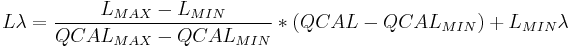$L\lambda = \frac{L_{MAX}λ - L_{MIN}λ}{QCAL_{MAX} - QCAL_{MIN}} * (QCAL - QCAL_{MIN}) + L_{MIN}\lambda$

where:
•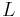$Lλ$ - spectral Radiance at the sensor's aperture in watts/(meter squared * ster * μm), the apparent radiance as seen by the satellite sensor ;
•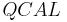$QCAL$ - the quantized calibrated pixel value in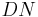$DN$ ;
•$LMINλ$ - the spectral radiance that is scaled to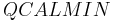$QCALMIN$ in watts/(meter squared * ster * μm) ;
•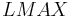$LMAXλ$ - the spectral radiance that is scaled to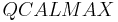$QCALMAX$ in watts/(meter squared * ster * μm) ;
•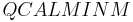$QCALMINM$ - the minimum quantized calibrated pixel value (corresponding to$LMINλ$) in$DN$ ;
•$QCALMAX$ - the maximum quantized calibrated pixel value (corresponding to$LMAXλ$) in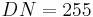$DN=255$.$LMINλ$ and$LMAXλ$ are the radiances related to the minimal and maximal$DN$ value, and are reported in the metadata file for each image, or in the table 1. High gain or low gain is also reported in the metadata file of each Landsat image. The minimal$DN$ value ($QCALMIN$) is 1 for Landsat ETM+ images (1), and the maximal$DN$ value ($QCALMAX$) is 255.$QCAL$ is the$DN$ value for every separate pixel in the Landsat image.

r.info lsat7_2002_xx


Under ‘comments’, the maximal and minimal radiance (LMAX and LMIN) for each band are given.

Power users may use this for nicely readable output (example for channel 1):

CHAN=1
r.info lsat7_2002_${CHAN}0 -h | tr '\n' ' ' | sed 's+ ++g' | tr ':' '\n' | grep "LMIN_BAND${CHAN}\|LMAX_BAND\${CHAN}"


Conversion to radiance (this calculation is done for band 1, for the other bands, the numbers in italics need to be replaced with their correct values):

g.region rast=lsat7_2002_10 -p
r.mapcalc "lsat7_2002_10_rad=((''191.6'' - (''-6.2'')) / (255.0 - 1.0)) * (lsat7_2002_10 - 1.0) + (''-6.2'')"


For faster computations, use an integer DEM:

 r.mapcalc "elev_int = round(elevation)"


Find mean elevation to initialize computations (needed in 'icnd' file below)

r.univar elev_int


## Estimating the overpass time

This value is needed for the control file. For details, refer to Wikipedia's entry on Scientific decimal time. In case this parameter is not reported in the metadata file, we have two ways to obtain it.

### From sun position

The satellite overpass time can be estimated rather precisely from the sun position reported in metadata using r.sunmask. The metadata file for the following example contains: SUN_AZIMUTH = 120.8810347, SUN_ELEVATION = 64.7730999.

# iteratively change hour and minutes to obtain a good result, timezone needs to be correct of course
r.sunmask  -s elev=elevation out=dummy year=2002 month=5 day=24 hour=10 min=42 sec=7 timezone=-5


The above command reports:

Solar position: sun azimuth: 121.342461, sun angle above horz.(refraction corrected): 65.396652


The resulting overpass local time 10:42:07 corresponds to 15:42 in GMT which corresponds to 15.70 in decimal GMT hours (decimal minutes: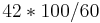$42 * 100 / 60$).

### From NASA web tool

The NASA LaRC Satellite Overpass Predictor can compute satellite overpass time from date of acquisition and scene center coordinates. The metadata file for the following example contains: ACQUISITION_DATE = 2002-05-24, SCENE_CENTER_LAT = +36.0512847, SCENE_CENTER_LON = -79.3280820.

1. Select the World zone in the bottom-right side of the page and click Go >>
2. Insert Lat/Long coordinates (decimal degrees, without plus sign but with minus sign)
3. Select the proper satellite
4. Insert the date of acquisition
5. Optional: select "Day" or "Night" to restrict computation to (local) day/night overpass of the satellite

## Atmospheric correction

Radiance values can be converted to reflectance values in undergoing an atmospheric correction by applying the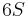$6S$ algorithm. The algorithm will transform the Top-of-Atmosphere radiance values to Top-of-Canopy reflectance values using predefined information on the aerosol content and atmospheric composition of the time the image was taken. What follows describes the method to use this algorithm in GRASS GIS. Again, only calculations for band 1 are demonstrated here, and the number(s) to be changed for indicating other spectral bands, are red. The icnd_lsat1.txt control file consists of the following parameters, and is written with a text editor:

8                          # indicates that it is an ETM+ image
05 24 15.67 -78.691 35.749 # image taken on the 24th of May, at 15:42 GMT in decimals; the center of the image lies at 78.691°W and 35.749°N
2                          # the midlatitude summer atmospheric model
1                          # the continental aerosol model
50                         # the visibility for the aerosol model [km]
-0.110                     # the terrain lies 110 meters above sea level [km] * -1
-1000                      # image taken of a satellite sensor (1000)
61                         # spectral band, here 1: blue


Alternatively, in case visibility maps are not available, an Aerosol Optical Depth estimation at 550nm can be used. The control file should be altered accordingly by entering 0 for the visibility and, in a following line, entering the aerosol optical depth. The control file should be structured as follows:

8                          # indicates that it is an ETM+ image
05 24 15.67 -78.691 35.749 # image taken on the 24th of May, at 15:42 GMT in decimals; the center of the image lies at 78.691°W and 35.749°N
2                          # the midlatitude summer atmospheric model
1                          # the continental aerosol model
0                          # set visibility = 0
0.112                      # e.g. aerosol optical depth
-0.110                     # the terrain lies 110 meters above sea level [km] * -1
-1000                      # image taken of a satellite sensor (1000)
61                         # spectral band, here 1: blue


This file is then used in the i.atcorr module:

i.atcorr -a -o iimg=lsat7_2002_10_rad ialt=elev_int icnd=icdn_lsat1.txt oimg=lsat7_2002_10_atcorr


Where:

• -a refers to a Landsat image taken after July 2000
• -o activates the cache acceleration
• iimg is the image to be corrected
• ialt is the altitude map which overrides the initialization value of 110 meters
• icnd is the path to the icnd.txt file
• oimg is the name of the output image

Detailed information can be found in i.atcorr's manual.

Note, i.atcorr wont complain if the defined elevation model (parameter elevation=) does not cover the extent of the Landsat scene that is the subject of the process -- it will result in NaN's. [Maybe this can be requested as an enhancement?]